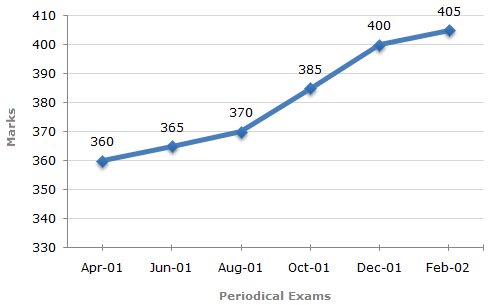# Data Interpretation - Line Charts - Discussion

### Discussion :: Line Charts - Line Chart 10 (Q.No.2)

In a school the periodical examination are held every second month. In a session during April 2001 - March 2002, a student of Class IX appeared for each of the periodical exams. The aggregate marks obtained by him in each perodical exam are represented in the line-graph given below.

Marks Obtained by student in Six Periodical Held in Every Two Months During the Year in the Session 2001 - 2002.

Maximum Total Marks in each Periodical Exam = 5002.

The total number of marks obtained in Feb. 02 is what percent of the total marks obtained in April 01 ?

 [A]. 110% [B]. 112.5% [C]. 115% [D]. 116.5%

Explanation:

Here it is clear from the graph that the student obtained 360, 365, 370, 385, 400 and 405 marks in periodical exams held in Apr 01, Jun 01, Aug 01, Oct 01, Dec 01 and Feb 02 respectively.

 Required percentage =405 x 100% = 112.5%. 360

 Kalita said: (Sep 9, 2014) 40/36 = (40*3)/(36*3) = 120/98 = approx. 12% +100 = 112.